Examples from NCERT Book

Class 9
Chapter 12 Class 9 - Sound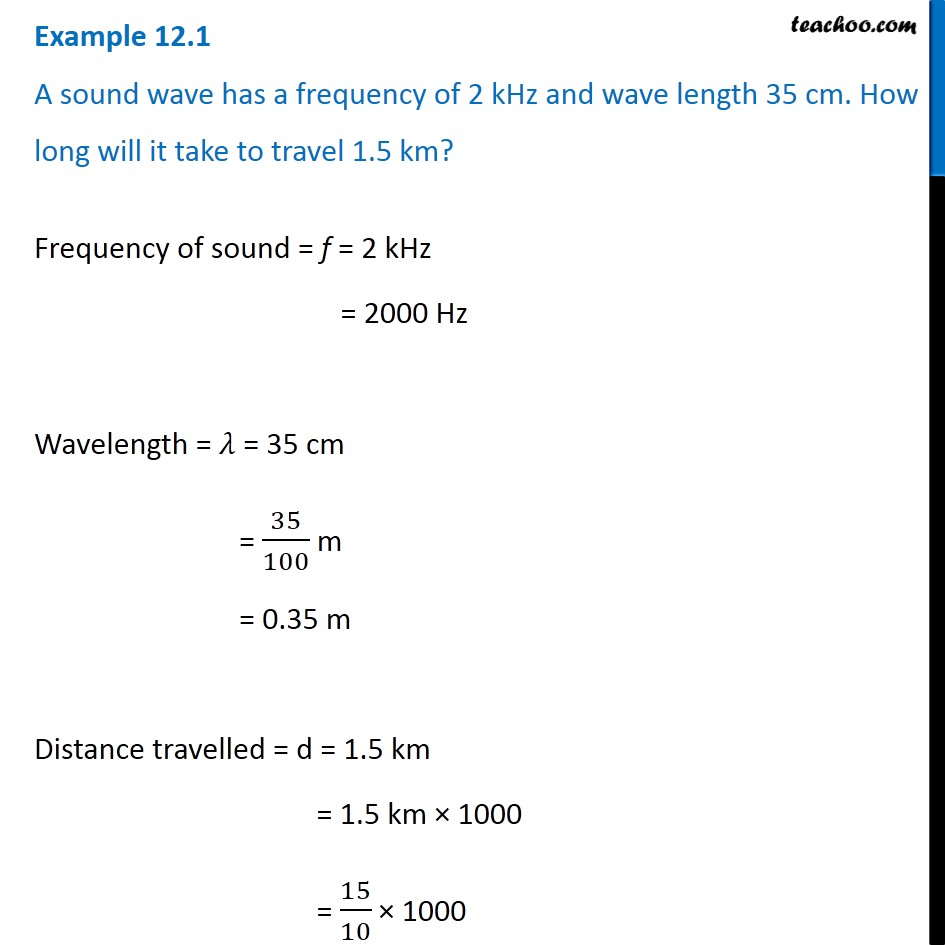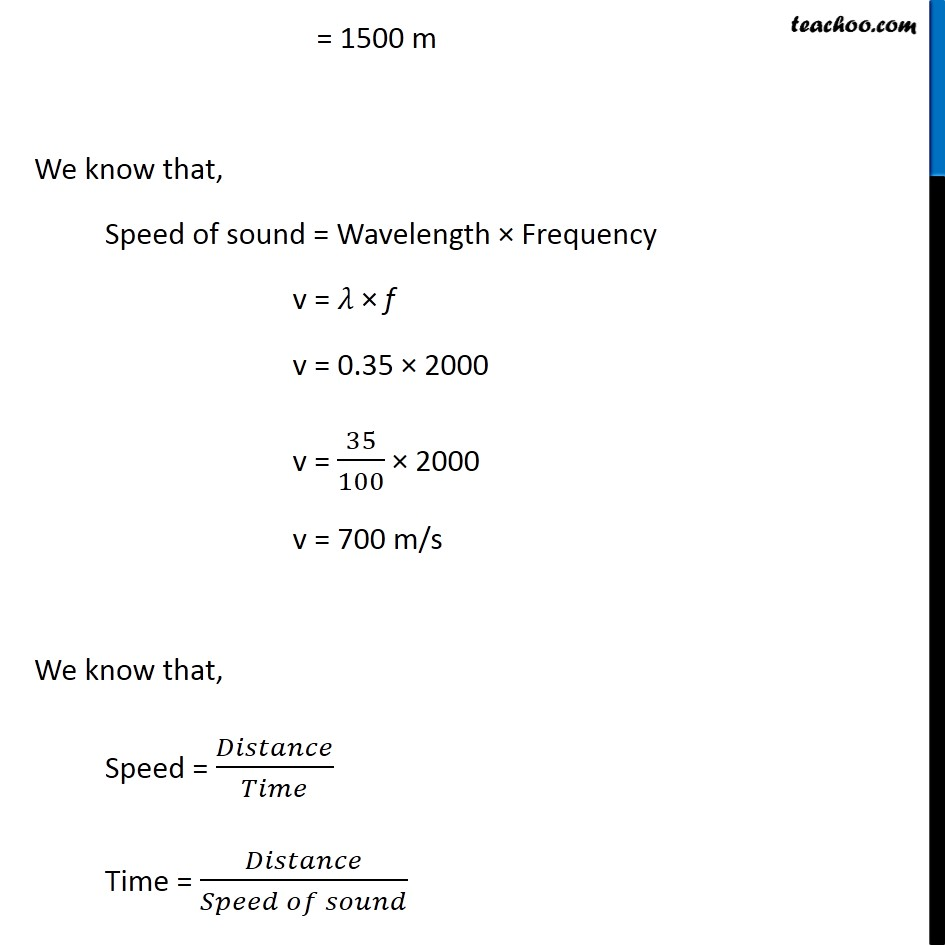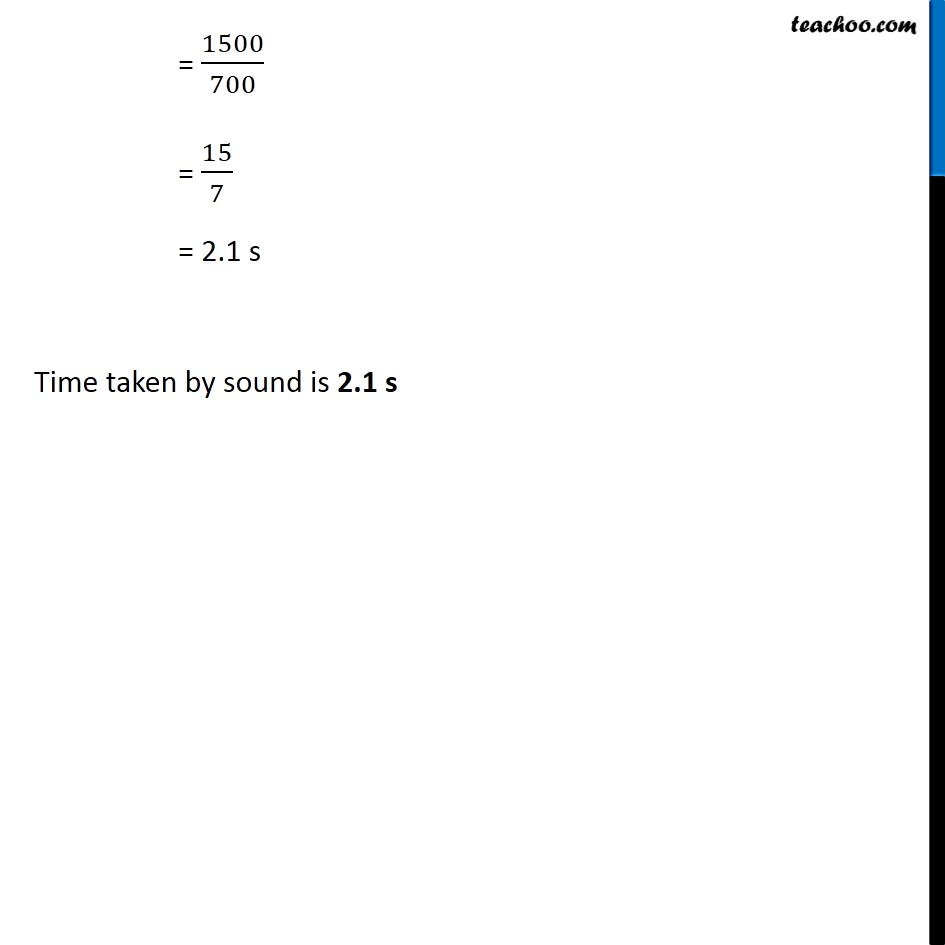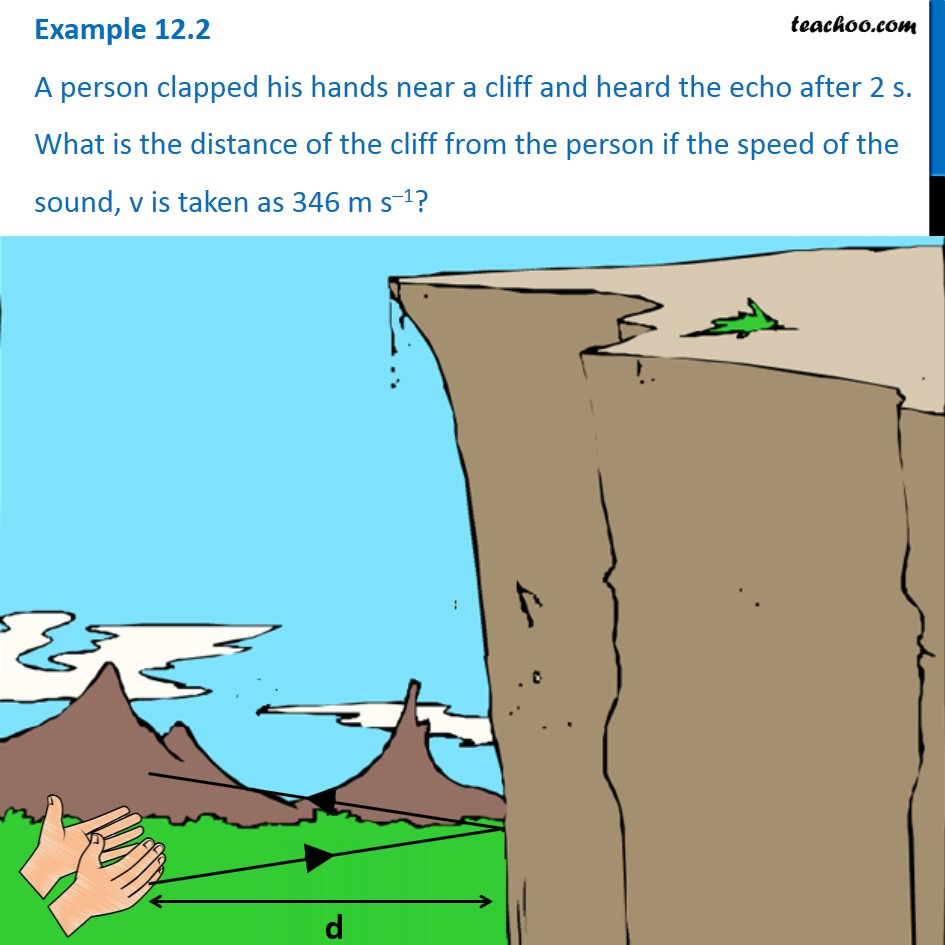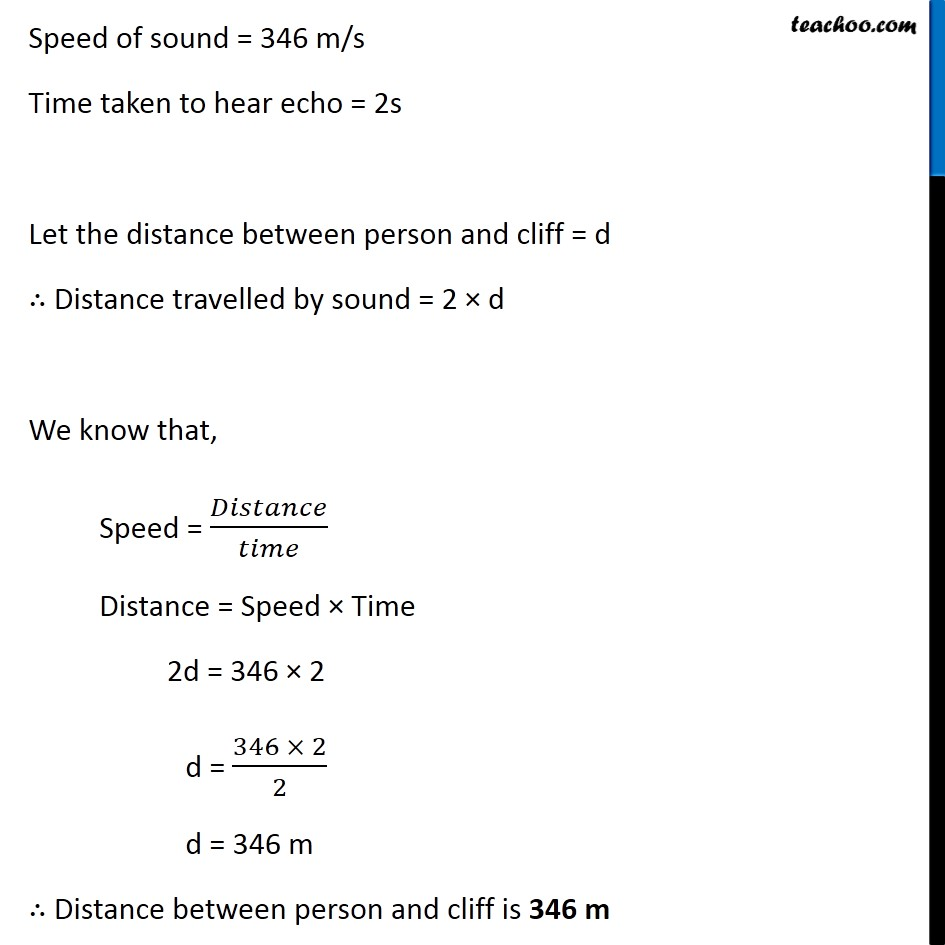Learn in your speed, with individual attention - Teachoo Maths 1-on-1 Class

### Transcript

Example 12.1 A sound wave has a frequency of 2 kHz and wave length 35 cm. How long will it take to travel 1.5 km? Frequency of sound = f = 2 kHz = 2000 Hz Wavelength = 𝜆 = 35 cm = 35/100 m = 0.35 m Distance travelled = d = 1.5 km = 1.5 km × 1000 = 15/10 × 1000 = 1500 m We know that, Speed of sound = Wavelength × Frequency v = 𝜆 × f v = 0.35 × 2000 v = 35/100 × 2000 v = 700 m/s We know that, Speed = 𝐷𝑖𝑠𝑡𝑎𝑛𝑐𝑒/𝑇𝑖𝑚𝑒 Time = 𝐷𝑖𝑠𝑡𝑎𝑛𝑐𝑒/(𝑆𝑝𝑒𝑒𝑑 𝑜𝑓 𝑠𝑜𝑢𝑛𝑑) = 1500/700 = 15/7 = 2.1 s Time taken by sound is 2.1 s